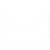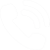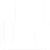## How Is the Center Frequency of a Monocycle Waveform Measured?

Avtech uses two methods to estimate the center frequency of a monocycle waveform. The first method is to measure the time between the positive and negative peaks of the waveform, and consider this time to be one-half of the period. The second method is to measure the time between the initial point where the output amplitude is 10% of the positive peak and the last point where the output amplitude is 10% of the negative peak, and consider this time to be equal to one period. The waveform below illustrates these techniques for one waveform.Using the peak-to-peak method, the center frequency can be estimated as:

fCENT = 1 / (2 x 0.45 ns) = 1.11 GHz

Using the 10%-to-10% method, the center frequency can be estimated as:

fCENT = 1 / (1.06 ns) = 0.945 GHz

These produce slightly different results, of course. Overall, the center frequency of the waveform can be estimated as ~ 1 GHz, using these two calculations. Intuitively, this seems correct, since the waveform fits almost entirely into a 1 ns wide area (one division on the oscilloscope photo), and 1 / 1 ns = 1 GHz.

Other methods can be used, of course, such as matching a "best-fit" curve of a particular mathematical equation to the waveform, or performing a Fourier transform, etc. However, since the actual waveform produced by monocycle rarely matches any ideal mathematical curve, Avtech uses the above "quick estimates" instead.### info@avtechpulse.com### ph. 888-670-8729 or +1-613-686-6675